# 3.1    3D Reconstruction with Implicit Surfaces

An implicit surface is defined by an implicit function which is a continuous function over the domain R 3. For example, a unit sphere may be defined using the implicit function f( p) = 1 ≤ | p |, for points p element of R 3. Points on the sphere are those locations at which f(p) = 0. A short introduction to implicit surfaces can be found in [ AMH02 ]. An important class of implicit surfaces are the ''blobby surfaces'' [ Bli82 ]. Such a surface is described by:
f( p) =
 n-1 ∑ i=0
h(r i)
where n is the number of primitive objects and for each of these objects a distance r i is computed, which is in example the distance from p to these objects. The blending function h describes the region of influence of object i. For spheres, r i is in example the distance from p to the center of sphere i.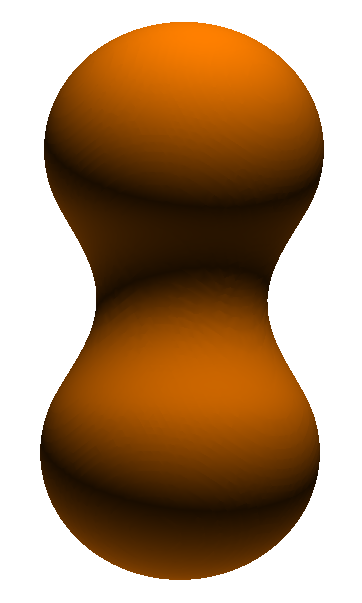Figure 1: Two blended spheres.

In this case, in the above equation, a single function h i describes the profile of a ''blobby sphere'' which is a Gaussian function that has a particular center and standard deviation. When the centers of two blobby spheres are close enough to one another, the implicit surface appears as though the two spheres have melted together.

Figure 1 shows two such blended spheres. All points on and between them, where they are blended together, are in the area of influence of the two centers and create the surface. The following WebGL link shows a corresponding 3D model: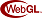.

To reconstruct the surface, a three-dimensional grid is placed over the area where the surface is located and the blending function is sampled at each grid point. A well-known method for creating a triangle mesh from such an implicit surface is the marching cubes [ LCl87 ] algorithm.

The following reconstruction examples (Figure 2) are based on the range data from the Stanford 3D Scanning Repository, used by courtesy of the Stanford Computer Graphics Laboratory. The rotation and translation information available with this range data has been used to move the parts (range images) together to form the starting point set. The information about mesh connectivity however has not been used for this examples. In this way the point set was used as unoriented point cloud.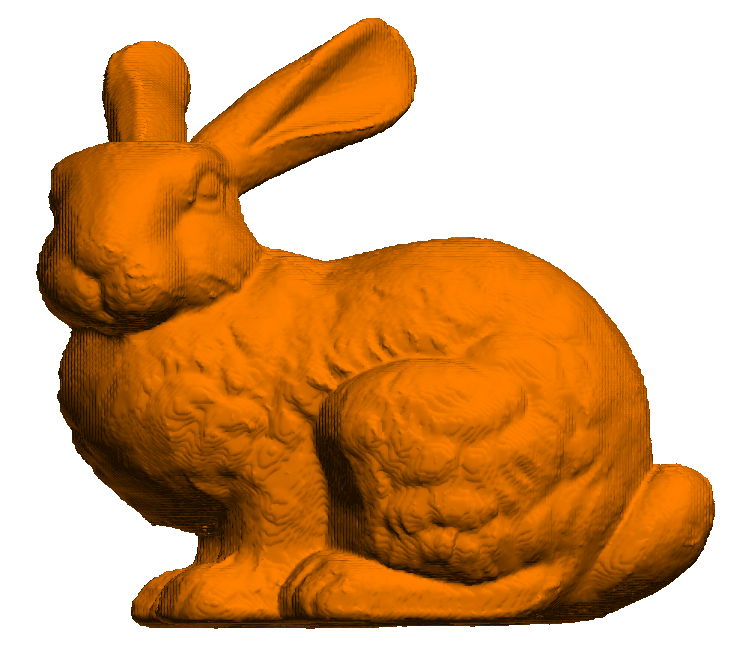(a) Bunny reconstructed from 362.272 points. (b) Dragon reconstructed from 1.235.765 points.
Figure 2: Reconstruction examples

Figure 3 shows the treatment of holes by different reconstruction approaches. What is best depends on the requirements of the application area. A hole is either intentional (in example drilled into the surface) or may be caused by low point density delivered by the scanning device. The bunny is shown from below where it has two holes and areas of low data density in the base plate.

Figure 3a is the result of Zipper [ TLe94 ]. The mesh produced by Zipper shows the holes and is not watertight.

The middle bunny (Figure 3b) is the one reconstructed with the above described implicit surface method. This bunny has a watertight mesh and shows the holes. It has a double sided surface.

For the right bunny (Figure 3c) the Poisson surface reconstruction [ KBH06 ] method has been used. This method does produce a watertight mesh with closed holes.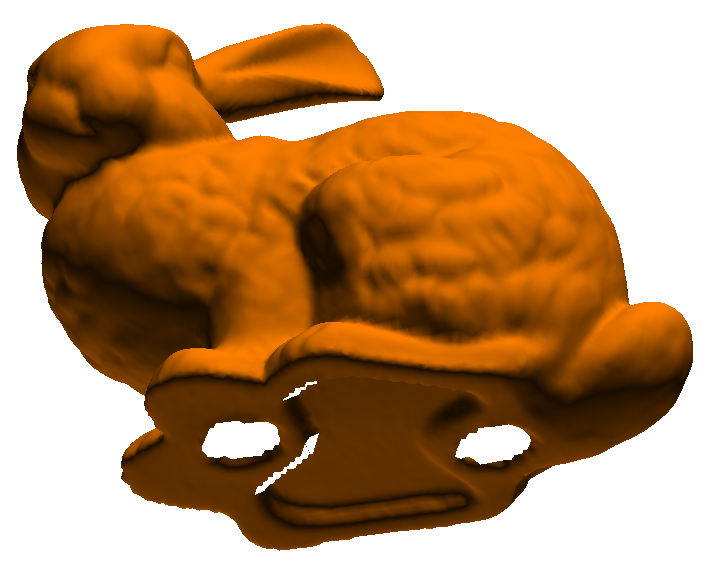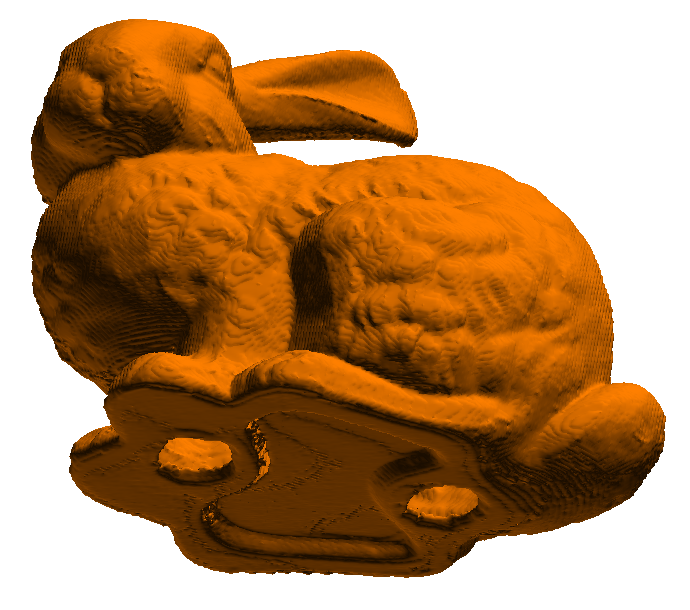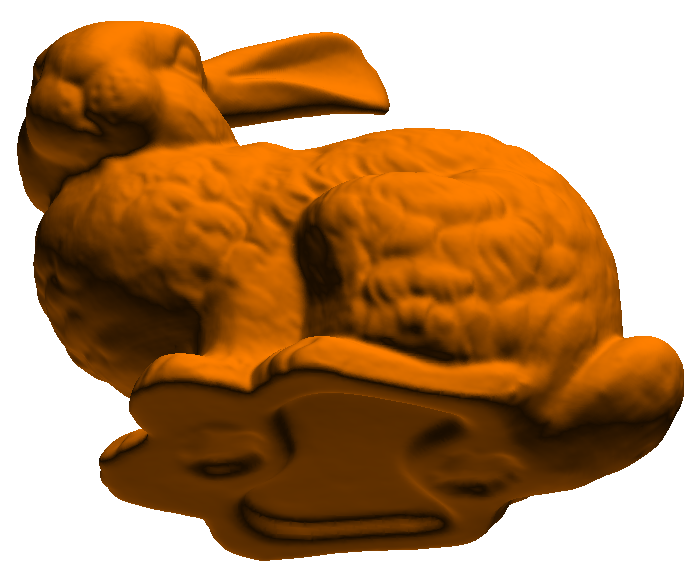(a) Zipper (b) Approach with implicit surfaces. (c) Poisson
Figure 3: Reconstruction examples

The following WebGL links show 3D models of the reconstruction examples. The mesh quality has been reduced to 35K/70 vertices/faces: Zipper, Approach with implicit surfacesand Poisson.